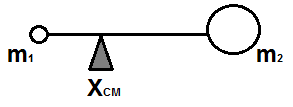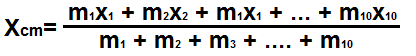﻿ Center of Mass Calculator ﻿# Center of Mass CalculatorM1: X1: M2: X1: M3: X3: M4: X4: M5: X5: M6: X6: M7: X7: M8: X8: M9: X9: M10: X10:

nan

This center of mass calculator calculates where the center of mass is located on an object.

An object can have many different masses distributed throughout it, at different distances along it (the object). The center of mass calculator calculates the distance, XCM, where along the object's length, there would be a balancing or equalization of mass from the two end points. This is like visualizing the effect of balancing a seesaw about a pivot given the two forces or masses present at both ends.

To use this calculator, the user enters in all the different masses present on throughout a given object at their various respective distances on the object. A user can enter up to 10 masses, with their respective distances, along the object, for this calculator. If a user just wants to enter, for example, 3 masses, then he or she just has to enter 3 values and leave the rest of the fields untouched.

Any unit can be used to represent XCM, including meters, feet, inches, etc. However, the standard and scientific unit would be meters (m).

The center of mass can be very useful in electronics because certain electronic components such as accelerometers make use of such concepts. An accelerometer is an electronic device which responds according to different forces upon it. Different masses, which cause different weights (w=mg), exert different forces (due to the differing masses). Thus, knowing the center of mass can come in handy and be very useful when dealing with electronic components such as this.

Example Calculations

Calculate the center of mass when the mass and distance of one item is 7kg and 5 meters and mass and distance of the second item is 10kg and 3 meters.

Center of Mass= (mass of item 1)(distance of item 1) + (mass of item 2)(distance of item 2)/(mass of item 1 + mass of item 2) = ((7)(5) + (10)(3))/(7+10)= (35+30)/(17)= 3.82.

So with one item having a mass of 7kg and being located at a distance of 5 meters and a second item having a mass of 10kg and being located at a distance of 3 meters, the center of mass is located at a distance of 3.82 meters.

Since the unit masses cancel out in the calculation, the resultant value is in whatever units the distance is entered in.

Second Example

Calculate the center of mass item 1 has a mass of 20kg and a distance of 1 meter, item 2 has a mass of 15kg at a distance of 7 meters, and item 3 has a mass of 25kg and a distance of 12 meters.

Center of Mass= (mass of item 1)(distance of item 1) + (mass of item 2)(distance of item 2)+ (mass of item 3)(distance of item 3)/ (mass of item 1 + mass of item 2 + mass of item 3) = ((20)(1) + (15)(7) + (25)(12))/(20 + 15 + 25)= (20 + 105 + 300 )/60= 425/60= 7.08.

So the center of mass is 7.08 meters.

Related Resources

﻿